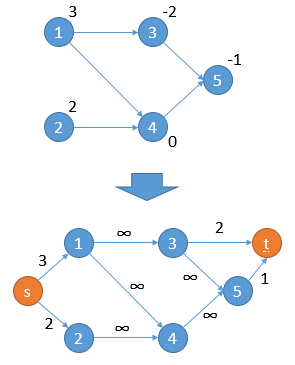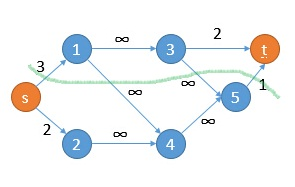# 定义

• 对于所有边$(u,v)$，点$u$在子图中则$v$必在子图中
• 子图的点权和最大

# 解法• 对于$w_i\gt 0$的点$i$，连边$(S,i,w_i)$
• 对于$w_i\lt 0$的点$i$，连边$(i,T,-w_i)$
• 对于原图边$(u,v)$，连边$(u,v,\infty)$

# 证明

• 最小割一定是简单割
• 简单割就是只割与$S$$T$相连的边
• 显然成立，因为原图边都是无穷大
• 简单割与闭合子图一一对应
• 闭合子图和$S$构成集合$V_S$，其余点和$T$构成集合$V_T$
• 下面是一个示例，$V_S=\{S,2,4,5\}$
•• 证明略

# 例题

• BZOJ1497 最大获利
• BZOJ1565 植物大战僵尸
• BZOJ4873 寿司餐厅